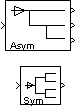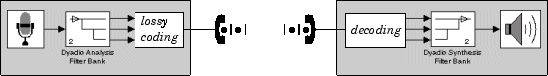Decompose signals into subbands with smaller bandwidths and slower sample rates or compute discrete wavelet transform (DWT)

## Library

Filtering / Multirate Filters

`dspmlti4`

•## Description

### Note

This block always interprets input signals as frames. The frame size of the input signal must be a multiple of 2n, where `n` is the value of the Number of levels parameter. The block decomposes the input signal into either n+1 or 2n subbands. To decompose signals with a frame size that is not a multiple of 2n, use the Two-Channel Analysis Subband Filter block. (You can connect multiple copies of the Two-Channel Analysis Subband Filter block to create a multilevel dyadic analysis filter bank.)

You can configure this block to compute the Discrete Wavelet Transform (DWT) or decompose a broadband signal into a collection of subbands with smaller bandwidths and slower sample rates. The block uses a series of highpass and lowpass FIR filters to repeatedly divide the input frequency range, as illustrated in Wavelet Filter Banks (the Asymmetric one).

You can specify the filter bank's highpass and lowpass filters by providing vectors of filter coefficients. You can do so directly on the block mask, or, if you have a Wavelet Toolbox™ license, you can specify wavelet-based filters by selecting a wavelet from the Filter parameter. You must set the filter bank structure to asymmetric or symmetric, and specify the number of levels in the filter bank.

For the same input, the DWT configuration of this block does not produce the same results as the Wavelet Toolbox `dwt` function. Because DSP System Toolbox™ is designed for real-time implementation and Wavelet Toolbox is designed for analysis, the products handle boundary conditions and filter states differently. To make the output of the `dwt` function match the DWT output of this block, complete the following steps:

1. Set the boundary condition of the `dwt` function to zero-padding. To do so, type `dwtmode('zpd')` at the MATLAB® command line.

2. To match the latency of the block (implemented using FIR filters), add zeros to the input of the `dwt` function. The number of zeros you add must be equal to the half-length of the filter.

### Input Requirements

• Input must be a vector or matrix.

• The input frame size must be a multiple of 2n, where n is the number of filter bank levels. For example, a frame size of 16 would be appropriate for a three-level tree (16 is a multiple of 23).

• The block always treats input signals as frames and operates along the columns.

For an illustration of why the above input requirements exist, see the figure Outputs of a 3-Level Asymmetric Dyadic Analysis Filter Bank.

### Output Characteristics

The output characteristics vary depending on the block's parameter settings, as summarized in the following list and figure:

• Number of levels parameter set to n

• Tree structure parameter setting:

• `Asymmetric` — Block produces n+1 output subbands

• `Symmetric` — Block produces 2n output subbands

• Output parameter setting can be ```Multiple ports``` or `Single port`. When you set the Output parameter to ```Single port```, the block outputs one vector or matrix of concatenated subbands. The following figure illustrates the difference between the two settings for a 3-level asymmetric dyadic analysis filter bank. For an explanation of the illustrated output characteristics, see the table Output Characteristics for an n-Level Dyadic Analysis Filter Bank.

Outputs of a 3-Level Asymmetric Dyadic Analysis Filter BankThe following table summarizes the different output characteristics of the block when it is set to output from single or multiple ports.

Output Characteristics for an n-Level Dyadic Analysis Filter Bank

Single Output PortMultiple Output Ports
Output Description

Block concatenates all the subbands into one vector or matrix, and outputs the concatenated subbands from a single output port. Each output column contains subbands of the corresponding input channel.

Block outputs each subband from a separate output port. The topmost port outputs the subband with the highest frequencies. Each output column contains a subband for the corresponding input channel.

Output Frame Rate

Not applicable

Same as input frame rate
(However, the output frame sizes can vary, so the output sample rates can vary.)

Output Dimensions (Frame Size)

Same number of rows and columns as the input.

The output has the same number of columns as the input. The number of output rows is the output frame size. For an input with frame size Mi output yk has frame size Mo,k:

• `Symmetric` — All outputs have the frame size, Mi / 2n.

• `Asymmetric` — The frame size of each output (except the last) is half that of the output from the previous level. The outputs from the last two output ports have the same frame size since they originate from the same level in the filter bank.

`${M}_{o,k}=\left\{\begin{array}{cc}{M}_{i}/{2}^{k}& \left(1\le k\le n\right)\\ {M}_{i}/{2}^{n}& \left(k=n+1\right)\end{array}$`

Output Sample Rate

Same as input sample rate.

Though the outputs have the same frame rate as the input, they have different frame sizes than the input. Thus, the output sample rates, Fso, k, are different from the input sample rate, Fsi:

• `Symmetric` — All outputs have the sample rate Fsi / 2n.

• `Asymmetric`

`${F}_{so,k}=\left\{\begin{array}{cc}{F}_{si}/{2}^{k}& \left(1\le k\le n\right)\\ {F}_{si}/{2}^{n}& \left(k=n+1\right)\end{array}$`

### Filter Bank Filters

You must specify the highpass and lowpass filters in the filter bank by setting the Filter parameter to one of the following options:

• `User defined` — Allows you to explicitly specify the filters with two vectors of filter coefficients in the Lowpass FIR filter coefficients and Highpass FIR filter coefficients parameters. The block uses the same lowpass and highpass filters throughout the filter bank. The two filters should be halfband filters, where each filter passes the frequency band that the other filter stops.

• Wavelet such as `Biorthogonal` or `Daubechies` — The block uses the specified wavelet to construct the lowpass and highpass filters using the Wavelet Toolbox `wfilters` function. Depending on the wavelet, the block might enable either the Wavelet order or Filter order [synthesis / analysis] parameter. (The latter parameter allows you to specify different wavelet orders for the analysis and synthesis filter stages.) You must have a Wavelet Toolbox license to use wavelets.

Specifying Filters with the Filter Parameter and Related Parameters

FilterSample Setting for Related Filter Specification ParametersCorresponding Wavelet Function Syntax

User-defined

Filters based on Daubechies wavelets with wavelet order `3`:

• Lowpass FIR filter coefficients =
```[0.0352 -0.0854 -0.1350 0.4599 0.8069 0.3327]```

• Highpass FIR filter coefficients =
```[-0.3327 0.8069 -0.4599 -0.1350 0.0854 0.0352] ```

None

Haar

None

`wfilters('haar')`

Daubechies

Wavelet order = `4`

`wfilters('db4')`

Symlets

Wavelet order = `3`

`wfilters('sym3')`

Coiflets

Wavelet order = `1`

`wfilters('coif1')`

Biorthogonal

Filter order [synthesis / analysis] = `[3/1]`

`wfilters('bior3.1')`

Reverse Biorthogonal

Filter order [synthesis / analysis] = `[3/1]`

`wfilters('rbio3.1')`

Discrete Meyer

None

`wfilters('dmey')`

## Examples

### Wavelets

The primary application for dyadic analysis filter banks and dyadic synthesis filter banks is coding for data compression using wavelets.

At the transmitting end, the output of the dyadic analysis filter bank is fed to a lossy compression scheme, which typically assigns the number of bits for each filter bank output in proportion to the relative energy in that frequency band. This represents the more powerful signal components by a greater number of bits than the less powerful signal components.At the receiving end, the transmission is decoded and fed to a dyadic synthesis filter bank to reconstruct the original signal. The filter coefficients of the complementary analysis and synthesis stages are designed to cancel aliasing introduced by the filtering and resampling.

See Calculate Channel Latencies Required for Wavelet Reconstruction for an example using the Dyadic Analysis and Dyadic Synthesis Filter Bank blocks.

### Examples

See the floating-point frame-based version of the DSP System Toolbox Wavelet Reconstruction and Noise Reduction example, which uses the Dyadic Analysis Filter Bank and Dyadic Synthesis Filter Bank blocks.

## Parameters

The parameters displayed in the block dialog vary depending on the setting of the Filter parameter. Only some of the parameters described below are visible in the dialog box at any one time.

Filter

The type of filter used to determine the high- and low-pass FIR filters in the filter bank:

Select `User defined` to explicitly specify the filter coefficients in the Lowpass FIR filter coefficients and Highpass FIR filter coefficients parameters.

Select a wavelet such as `Biorthogonal` or `Daubechies` to specify a wavelet-based filter. The block uses the Wavelet Toolbox `wfilters` function to construct the filters. Extra parameters such as Wavelet order or Filter order [synthesis / analysis] might become enabled. For a list of the supported wavelets, see Specifying Filters with the Filter Parameter and Related Parameters.

Lowpass FIR filter coefficients

A vector of filter coefficients (descending powers of z) that specifies coefficients used by all the lowpass filters in the filter bank. This parameter is enabled when you set Filter to `User defined`. The lowpass filter should be a half-band filter that passes the frequency band stopped by the filter specified in the Highpass FIR filter coefficients parameter. The default values of this parameter specify a filter based on a Daubechies wavelet with wavelet order `3`.

Highpass FIR filter coefficients

A vector of filter coefficients (descending powers of z) that specifies coefficients used by all the highpass filters in the filter bank. This parameter is enabled when you set Filter to `User defined`. The highpass filter should be a half-band filter that passes the frequency band stopped by the filter specified in the Lowpass FIR filter coefficients parameter. The default values of this parameter specify a filter based on a Daubechies wavelet with wavelet order `3`.

Wavelet order

The order of the wavelet selected in the Filter parameter. This parameter is enabled only when you set Filter to certain types of wavelets, as shown in the Specifying Filters with the Filter Parameter and Related Parameters table.

Filter order [synthesis / analysis]

The order of the wavelet for the synthesis and analysis filter stages. For example, when you set the Filter parameter to `Biorthogonal` and set the Filter order [synthesis / analysis] parameter to ```[2 / 6]```, the block calls the `wfilters` function with input argument `'bior2.6'`. This parameter is enabled only when you set Filter to certain types of wavelets, as shown in Specifying Filters with the Filter Parameter and Related Parameters.

Number of levels

The number of filter bank levels. An n-level asymmetric structure has n+1 outputs, and an n-level symmetric structure has 2n outputs, as shown in Wavelet Filter Banks. The block's icon changes depending on the value of this parameter.

The default setting of this parameter is `2`.

Tree structure

The structure of the filter bank: `Asymmetric`, or `Symmetric`. See Wavelet Filter Banks.

The default setting of this parameter is `Asymmetric` for the Dyadic Analysis Filter Bank block, and `Symmetric` for the DWT block.

Output

Set to `Multiple ports` to output each output subband on a separate port (the topmost port outputs the subband with the highest frequency band). Set to `Single port` to concatenate the subbands into one vector or matrix and output the concatenated subbands on a single port. For more information, see Output Characteristics.

The default setting of this parameter is ```Multiple ports``` for the Dyadic Analysis Filter Bank block, and `Single port` for the DWT block.

## References

Fliege, N. J. Multirate Digital Signal Processing: Multirate Systems, Filter Banks, Wavelets. West Sussex, England: John Wiley & Sons, 1994.

Strang, G. and T. Nguyen. Wavelets and Filter Banks. Wellesley, MA: Wellesley-Cambridge Press, 1996.

Vaidyanathan, P. P. Multirate Systems and Filter Banks. Englewood Cliffs, NJ: Prentice Hall, 1993.

## Supported Data Types

PortSupported Data Types

Input

• Double-precision floating point

• Single-precision floating point

Output

• Double-precision floating point

• Single-precision floating point